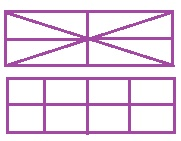Home | | Maths 4th Std | Exercise 6.1 (natural fractions)

# Exercise 6.1 (natural fractions)

Text Book Back Exercises Questions with Answers, Solution : 4th Maths : Term 2 Unit 6 : Fraction : Exercise 6.1 (Introduction to natural fractions)

Exercise 6.1

1. Observe the following pictures and write how many equal parts are they divided into ?2. Draw square and circle. Make it into four equal parts.3. Draw a rectangle and divide it into eight equal parts.Tags : Fraction | Term 2 Chapter 6 | 4th Maths , 4th Maths : Term 2 Unit 6 : Fraction
Study Material, Lecturing Notes, Assignment, Reference, Wiki description explanation, brief detail
4th Maths : Term 2 Unit 6 : Fraction : Exercise 6.1 (natural fractions) | Fraction | Term 2 Chapter 6 | 4th Maths